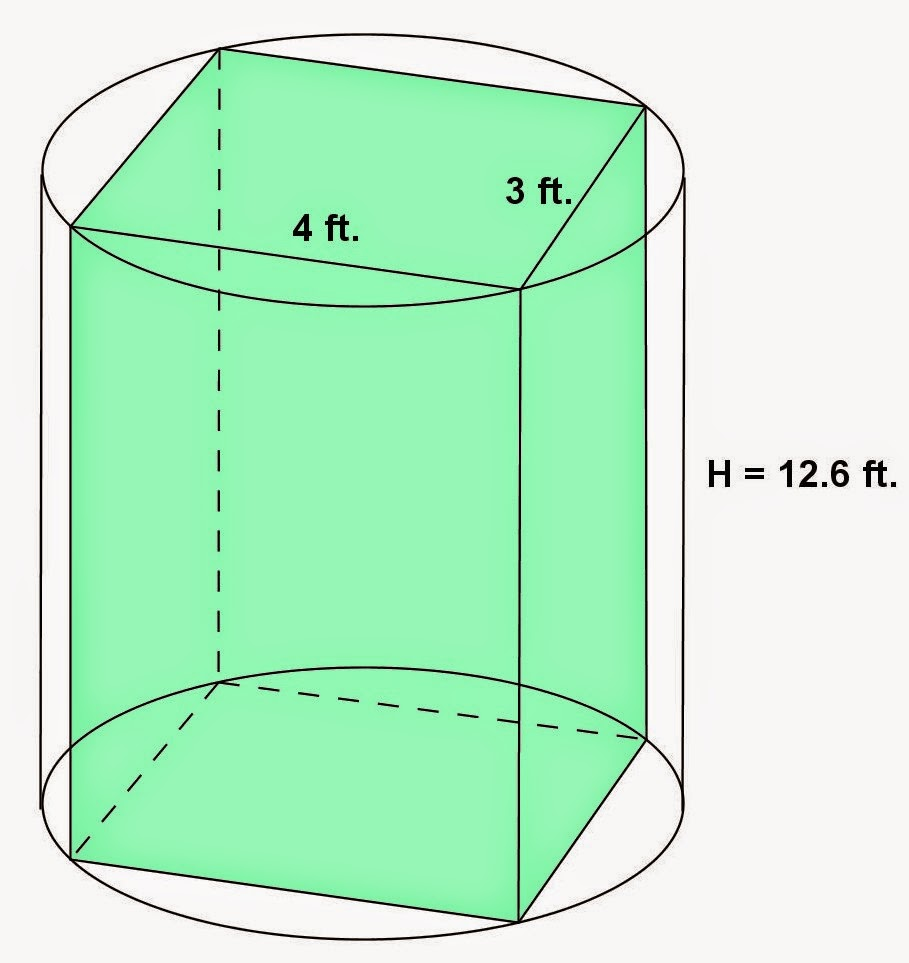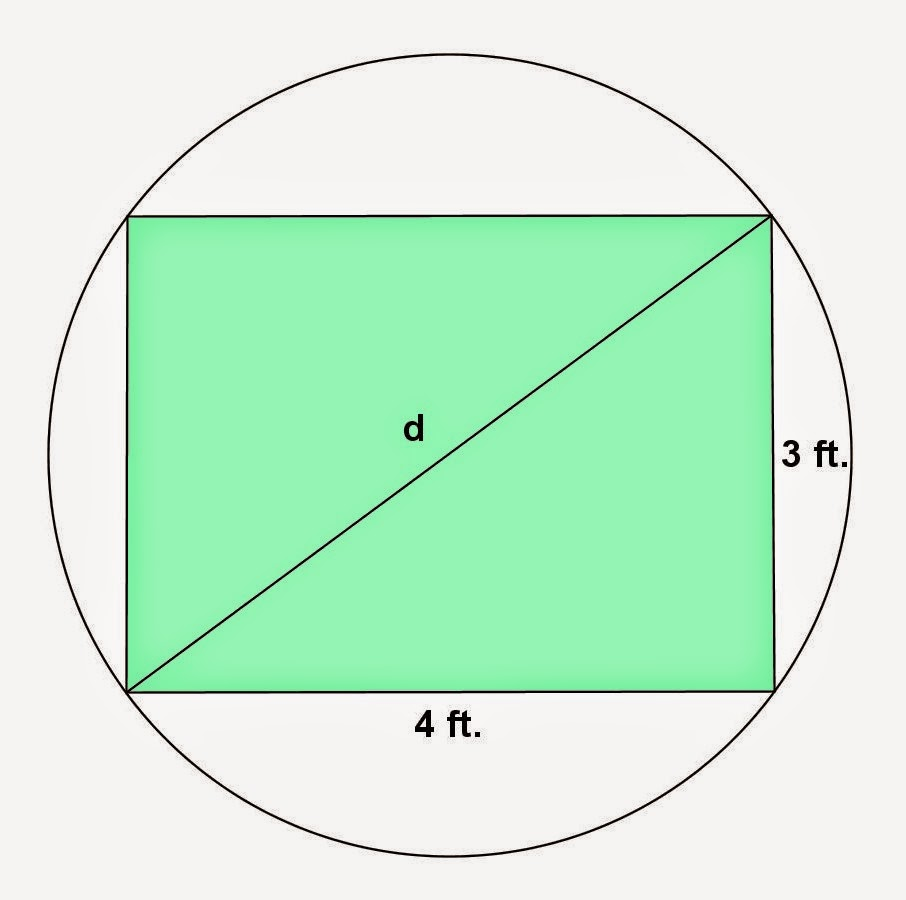## Sunday, August 31, 2014

### More Cylinder Problems, 11

Category: Solid Geometry

"Published in Vacaville, California, USA"

A cylinder whose base is a circle is circumscribed about a right prism of altitude 12.6 ft. Find the volume of the cylinder if the base of the prism is an equilateral triangle of side 4 ft.

Solution:

To illustrate the problem, it is better to draw the figure as followsPhoto by Math Principles in Everyday LIfe

Consider the base of a cylinder which is a circle that circumscribed about an equilateral triangle as followsPhoto by Math Principles in Everyday Life

The radius of a circle is equal to two thirds of the altitude of an equilateral triangle. By Pythagorean Theorem, the altitude of an equilateral triangle is

Hence, the radius of a circle is

Therefore, the volume of a circular cylinder is

But

Hence, the above equation becomes

or

## Saturday, August 30, 2014

### More Cylinder Problems, 10

Category: Solid Geometry

"Published in Vacaville, California, USA"

A cylinder whose base is a circle is circumscribed about a right prism of altitude 12.6 ft. Find the volume of the cylinder if the base of the prism is a rectangle 3 ft. by 4ft.

Solution:

To illustrate the problem, it is better to draw the figure as followsPhoto by Math Principles in Everyday Life

Consider the base of a cylinder which is a circle that circumscribed about a rectangle as followsPhoto by Math Principles in Everyday Life

The length of the diagonal of a rectangle is equal to the diameter of a circle. By Pythagorean Theorem, the diameter of a circle is

Therefore, the volume of a circular cylinder is

But

Hence, the above equation becomes

or

## Friday, August 29, 2014

### More Cylinder Problems, 9

Category: Solid Geometry

"Published in Newark, California, USA"

A cylinder whose base is a circle is circumscribed about a right prism of altitude 12.6 ft. Find the volume of the cylinder if the base of the prism is a square of edge 3 ft.

Solution:

To illustrate the problem, it is better to draw the figure as followsPhoto by Math Principles in Everyday Life

Consider the base of a cylinder which is a circle that circumscribed about a square as followsPhoto by Math Principles in Everyday Life

The length of the diagonal of a square is equal to the diameter of a circle. By Pythagorean Theorem, the diameter of a circle is

Therefore, the volume of a circular cylinder is

But

Hence, the above equation becomes

or